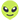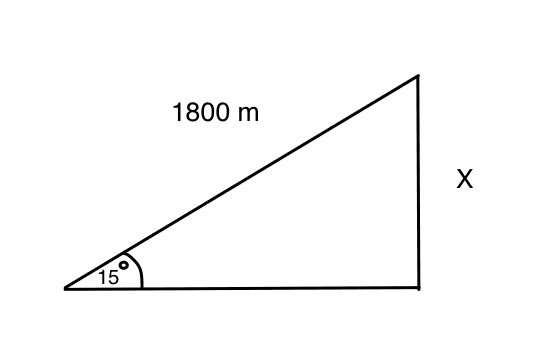# Power of a bicycle accelerating up a slope.

No template because originally posted in wrong thread
Hello everyoneI have been assigned to create a physics problem involving bicycles. I thought up the following, but I had questions regarding if it was properly made / worked out.

A cyclist is accelerating constantly up a slope at 1 m/s ^-2 The slope is at a 15 degree angle and is 30 m long. It takes the cyclist 1 minute to reach the end of the slope. The combined mass of the cyclist and the bicycle is 80 kg. How much power is needed to make this possible ? (Neglect air resistance and drag)

I was thinking of using the formula: F = mg sin θ + ma to calculate the force needed to go up the slope, and then incorporating the found value of F into the formula: P = work / time = ( F x d ) / t to find the amount power needed to complete this task. The working out looks like this:

P = ( ( mg sin θ + ma ) x d ) / t
P = ( (( 80 x 9.81 ) sin ( 15 ) + ( 80 x 1 )) 30 ) / 60
P = 141.6 W

Is this correct ? Are there any variables of e.g. vectors, forces, etc. that I have forgotten to include in this calculation? or am I over complicating things ?

Thank you very much !

Chestermiller
Mentor
How do you know that after 1 minute, the bicycle only covers 30 m? I get 1800 m (almost 2 km). The work is also calculated incorrectly. The work is equal to the change in potential energy plus the change in kinetic energy. Also, the power is not constant. The work divided by the time is equal to the average power.

How do you know that after 1 minute, the bicycle only covers 30 m? I get 1800 m (almost 2 km). The work is also calculated incorrectly. The work is equal to the change in potential energy plus the change in kinetic energy. Also, the power is not constant. The work divided by the time is equal to the average power.

Thank you for making me notice that ! At the time, I was just trying to make up some figures off the top of my head, but now I realise I had to use the formula:
d = 1/2 a t^2 to figure out the distance traveled at that acceleration.
I also appreciate the correction on the work done formula. If I'm correct, it should look like this now :

W = Δ Ep + Δ Ek
____________________________________________________________________________________________

ΔEp = mg Δh
= ( 80 ) ( 9.81 ) Δ( length of X = 465.9 m - see right angled triangle )
= 319915.8 JSOH
X = sin(15) x 1800 = 465.9 m
___________________________________________________________________________________________

ΔEk = Ek f - Ek i
= (1/2 m (v final)^2) - (1/2 m (v initial)^2)
v initial = 0
v final = (v initial) + at = (0) + (1)(60) = 60 m.s^-1
= (1/2 (80) (60)^2) - (1/2 (80) (0)^2)
= 144000 J
___________________________________________________________________________________________
W = (319915.8) + (144000)
= 463,915 .8 J

Average Power = work / time

Av P = ( 463,915.8) / 60
= 7731.9 W
____________________________________________________________________________________

However, upon seeing the final answer I cant help but feel like I miscalculated something ( I suspect an error when calculating the change in kinetic energy, or maybe the scenario simply isn't realistic ) because the magnitude of the answer - I feel - is too big for a cyclist.

Thank you for your feedback !

billy_joule
However, upon seeing the final answer I cant help but feel like I miscalculated something ( I suspect an error when calculating the change in kinetic energy, or maybe the scenario simply isn't realistic ) because the magnitude of the answer - I feel - is too big for a cyclist.

The scenario isn't realistic, cyclists don't cycle at over 200km/hr (60m/s) and can't accelerate at a constant rate. They are close enough to constant power device, like a car. A quick google says an elite cyclist can maintain ~500W over an hour, ~1,200W in short bursts.

•Delta2
The scenario isn't realistic, cyclists don't cycle at over 200km/hr (60m/s) and can't accelerate at a constant rate. They are close enough to constant power device, like a car. A quick google says an elite cyclist can maintain ~500W over an hour, ~1,200W in short bursts.
Thank you !

CWatters
Homework Helper
Gold Member
Perhaps simplify the problem by making it constant velocity rather than constant acceleration? or would that be too easy?

Perhaps turn the problem on it's head. The max power a cyclist can generate is 300W, how fast can he go up the slope?

How about a problem involving a unicyclist and working out the angle he must lean forwards or something like that?

•Chestermiller
Mentor
You can still use the problem as stated, but you have some input numbers that you can play with to make the problem more realistic: the angle, the time, the acceleration. Play around with these until you get a more realistic power value.

Perhaps simplify the problem by making it constant velocity rather than constant acceleration? or would that be too easy?

Perhaps turn the problem on it's head. The max power a cyclist can generate is 300W, how fast can he go up the slope?

How about a problem involving a unicyclist and working out the angle he must lean forwards or something like that?

Thats a very good idea! Thanks for helping me out.

You can still use the problem as stated, but you have some input numbers that you can play with to make the problem more realistic: the angle, the time, the acceleration. Play around with these until you get a more realistic power value.

Thank you! Ill toy around with the equation a bit